# barh

Horizontal bar graph

•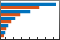## Syntax

``barh(y)``
``barh(x,y)``
``barh(___,width)``
``barh(___,style)``
``barh(___,color)``
``barh(___,Name,Value)``
``barh(ax,___)``
``b = barh(___)``

## Description

example

````barh(y)` creates a horizontal bar graph with one bar for each element in `y`. If `y` is an m-by-n matrix, then `barh` creates m groups of n bars.```

example

````barh(x,y)` draws the bars along the vertical axis at the locations specified by `x`.```

example

````barh(___,width)` specifies the fraction of available space occupied by each bar. For example, `barh(y,1)` makes the bars in each group touch each other. Specify `width` as the last argument in any of the previous syntaxes.```

example

````barh(___,style)` specifies the style of the bar groups. For example, `barh(y,'stacked')` stacks the bars within each group into a multicolored bar.```

example

````barh(___,color)` specifies a single color for all the bars. For example, `barh(y,'red')` displays red bars.```

example

````barh(___,Name,Value)` specifies properties of the bar graph using one or more name-value pair arguments. Only bar graphs that use the default `'grouped'` or `'stacked'` style support setting bar properties. Specify the name-value pair arguments after all other input arguments. For a list of properties, see Bar Properties.```

example

````barh(ax,___)` displays the bar graph in the target axes. Specify the axes as the first argument in any of the previous syntaxes.```

example

````b = barh(___)` returns one or more `Bar` objects. If `y` is a vector, then `barh` returns one `Bar` object. If `y` is a matrix, then `barh` returns a `Bar` object for each series. Use `b` to set properties of the bars after displaying the bar graph.```

## Examples

collapse all

Create a vector of four values. Display the values in a bar graph with one horizontal bar for each value.

```y = [10 20 30 41]; barh(y)```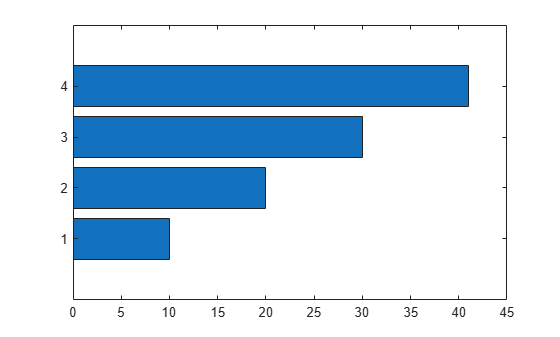Define `x` as a matrix of three year values. Define `y` as a matrix containing snowfall data for four cities. Display the four series of bars in groups for each year. Then add the axis labels and a legend.

```x = [1980 1990 2000]; y = [40 50 63 52; 42 55 50 48; 30 20 44 40]; barh(x,y) xlabel('Snowfall') ylabel('Year') legend({'Springfield','Fairview','Bristol','Jamesville'})```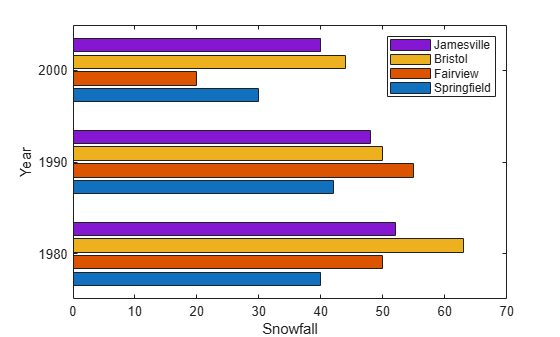Create matrix `y`. Then display the values of `y` in a bar graph with a baseline value of `25`. Values that are less than `25` display on the left side of the baseline.

```y = [8 15 33; 30 35 40; 50 55 62]; barh(y,'BaseValue',25)```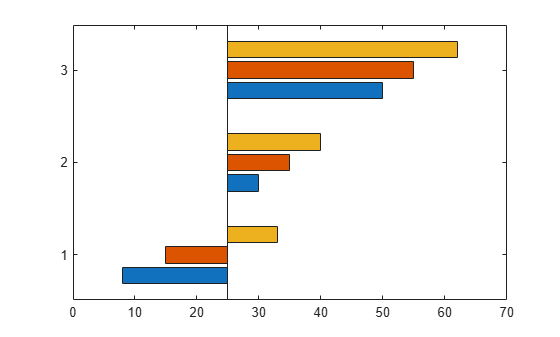Define `x` as a vector of three year values. Define `y` as a matrix that contains a combination of negative and positive values. Display the values in a stacked horizontal bar graph.

```x = [1980 1990 2000]; y = [15 20 -5; 10 -17 21; -10 5 15]; barh(x,y,'stacked')```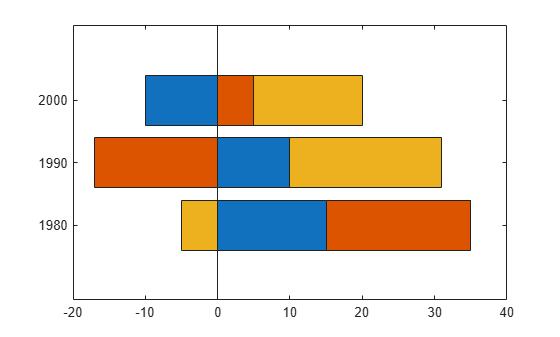Define `y` as a vector of four values, and display the values in a horizontal bar graph. Then call the `yticklabels` function to change the tick labels on the vertical axis.

```y = [10 20 30 41]; barh(y) yticklabels({'April','May','June','July'})```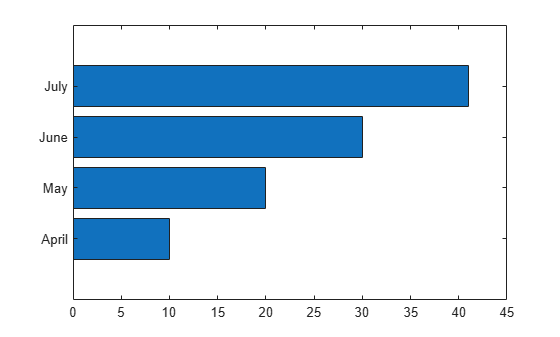One way to indicate categories for your bars is to specify `X` as a categorical array. The `barh` function uses a sorted list of the categories, so the bars might display in a different order than you expect. To preserve the order, call the `reordercats` function.

Define `X` as categorical array, and call the `reordercats` function to specify the order for the bars. Then define `Y` as a vector of bar lengths and display the bar graph.

```X = categorical({'Small','Medium','Large','Extra Large'}); X = reordercats(X,{'Small','Medium','Large','Extra Large'}); Y = [10 21 33 52]; barh(X,Y)```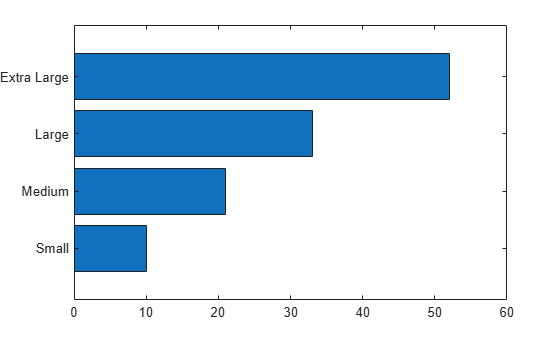Define `vals` as a matrix containing two series of data. Display the data in a horizontal bar graph and specify an output argument. Since there are two series, `barh` returns a vector of two `Bar` objects.

```x = [1 2 3]; vals = [2 3 6; 11 23 26]; b = barh(x,vals);```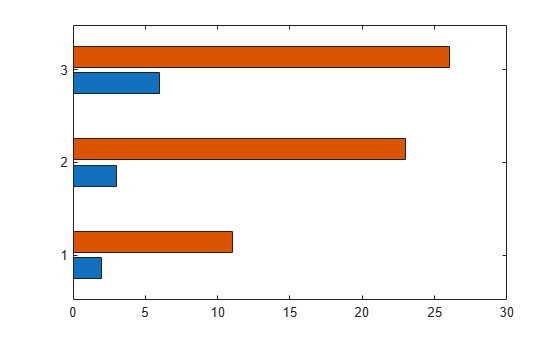Display the values as labels at the tips of the first series of bars. To do this, get the coordinates of the tips of the bars by getting the `XEndPoints` and `YEndPoints` properties of the first `Bar` object. Since horizontal bar graphs have rotated axes, you must switch the values of `XEndPoints` and `YEndPoints` before passing them to the `text` function. Add a padding value of `0.3` to `YEndpoints` so that the text does not touch the edges of the bars. Then call the `text` function to display the labels.

```xtips1 = b(1).YEndPoints + 0.3; ytips1 = b(1).XEndPoints; labels1 = string(b(1).YData); text(xtips1,ytips1,labels1,'VerticalAlignment','middle')```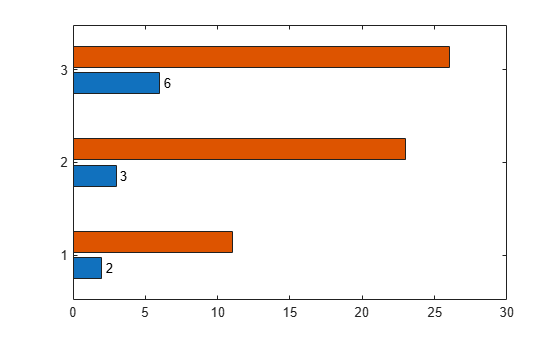Next, follow the same steps to display the labels at the tips of the second series of bars.

```xtips2 = b(2).YEndPoints + 0.3; ytips2 = b(2).XEndPoints; labels2 = string(b(2).YData); text(xtips2,ytips2,labels2,'VerticalAlignment','middle')```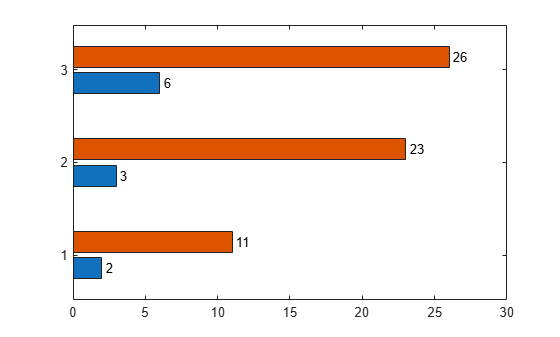Create and display data in a horizontal bar graph that has red bars with a thickness of `0.4`.

```y = [10 22 30 42]; width = 0.4; barh(y,width,'red');```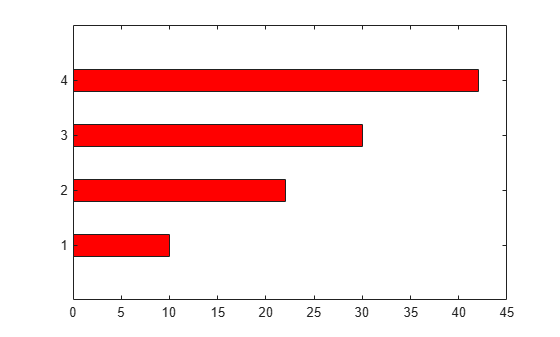Create matrix `y` in which each column is a series of data. Then display the data in a bar graph, specifying an output argument when calling the `barh` function. In this case, `barh` returns a vector of three `Bar` objects. Each object corresponds to a different series.

```y = [10 15 20; 30 35 40; 50 55 62]; b = barh(y);```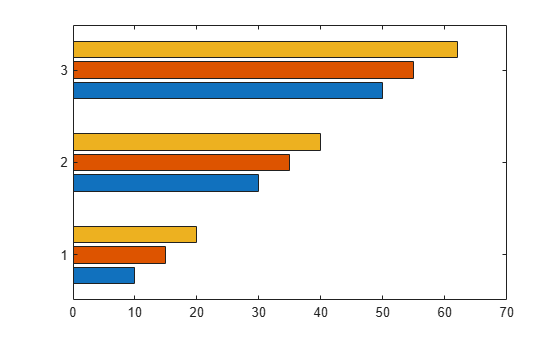Modify the second series of bars to be green with thick red edges.

```b(2).FaceColor = [.2 .6 .5]; b(2).EdgeColor = [.63 .08 .18]; b(2).LineWidth = 2;```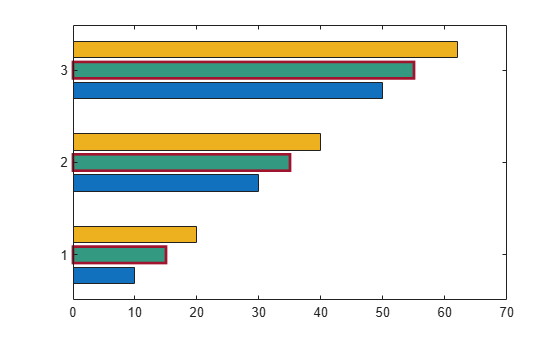Create and display data in two different bar graphs using the default `'grouped'` style and the `'stacked'` style.

```x = [1980 1990 2000]; y = [8 15 25; 30 35 40; 50 55 62]; % Grouped tiledlayout(2,1); ax1 = nexttile; barh(ax1,x,y) title('Grouped Style') % Stacked ax2 = nexttile; barh(ax2,x,y,'stacked') title('Stacked Style')```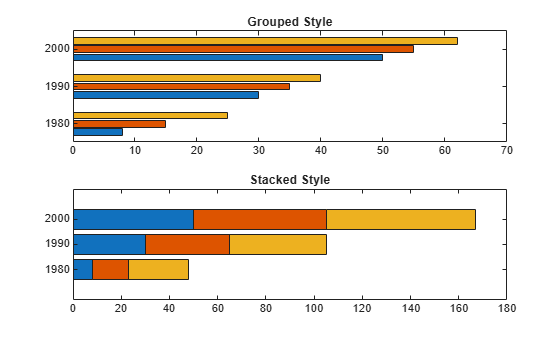## Input Arguments

collapse all

Vertical axis coordinates, specified as a scalar, vector, or matrix. The values of `x` do not need to be in order, but the size of `x` depends on the size of `y` and how you want to display your data. This table describes the most common situations.

PresentationHow to Specify `X` and `Y`Example
Display one series of bars.

Specify `x` and `y` as vectors that are the same length. The values in `x` must be unique, but the values in `y` do not need to be unique.

```x = [1980 1990 2000]; y = [10 20 30]; barh(x,y)```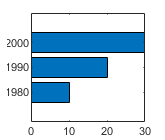Display multiple series of bars in groups.

Specify either of these combinations:

• Specify `x` and `y` as matrices of equal size. Each column of `y` corresponds to a series of bars. By default, each series is a different color. To ensure consistent placement of the groups, specify the columns of `x` as identical vectors. The values within a column must be unique, even though the columns are repeated.

• Specify `x` as a vector of unique values, and specify `y` as a matrix. The length of `x` must equal the length of at least one dimension of `y`. The other dimension of `y` contains values for the different series of bars.

```x = [1980 1980 1980 1990 1990 1990]; y = [2 6 9 11 22 32]; barh(x,y)```
Or
```x = [1980 1990]; y = [2 6 9 11 22 32]; barh(x,y)```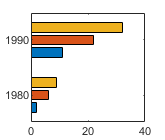Display one group of bars centered at one `x` value.

Specify `x` as a scalar and `y` as a vector.

```x = 1990; y = [10 20 30]; barh(x,y)```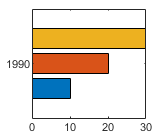Data Types: `single` | `double` | `int8` | `int16` | `int32` | `int64` | `uint8` | `uint16` | `uint32` | `uint64` | `categorical` | `datetime` | `duration`

Bar lengths, specified as a scalar, vector, or matrix. The size of `y` depends on the size of `x` and how you want to display your data. This table describes the most common situations.

PresentationHow to Specify `X` and `Y`Example
Display one series of bars.

Specify `x` and `y` as vectors that are the same length. The values in `x` must be unique, but the values in `y` do not need to be unique.

```x = [1980 1990 2000]; y = [10 20 30]; barh(x,y)```Display multiple series of bars in groups.

Specify either of these combinations:

• Specify `x` and `y` as matrices of equal size. Each column of `y` corresponds to a series of bars. By default, each series is a different color. To ensure consistent placement of the groups, specify the columns of `x` as identical vectors. The values within a column must be unique, even though the columns are repeated.

• Specify `x` as a vector of unique values, and specify `y` as a matrix. The length of `x` must equal the length of at least one dimension of `y`. The other dimension of `y` contains values for the different series of bars.

```x = [1980 1980 1980 1990 1990 1990]; y = [2 6 9 11 22 32]; barh(x,y)```
Or
```x = [1980 1990]; y = [2 6 9 11 22 32]; barh(x,y)```Display one group of bars centered at one `x` value.

Specify `x` as a scalar and `y` as a vector.

```x = 1990; y = [10 20 30]; barh(x,y)```Data Types: `single` | `double` | `int8` | `int16` | `int32` | `int64` | `uint8` | `uint16` | `uint32` | `uint64` | `duration`

Bar thickness, specified as a fraction of the total space available for each bar. The default of `0.8` means each bar uses 80% of the space from the previous bar to the next bar, with 10% of empty space on either side.

If the thickness is `1`, then the bars within a group touch one another.

Example: `barh([1 2 3],0.5)` creates bars that use 50% of the available space.

Group style, specified by one of these values.

StyleResultExample

`'grouped'`

Display each group as adjacent bars that are centered around the corresponding `x` value.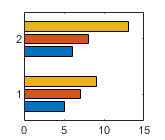`'stacked'`

Display each group as one multicolored bar. The length of a bar is the sum of the elements in the group.

If `y` is a vector, then the result is the same as `'grouped'`.`'histc'`

Display the bars in histogram format, in which the bars in a group touch one another. The trailing edge of each group is aligned with the corresponding `x` value.

Note

A better way to display a horizontal histogram is to call the `histogram` function and specify the `Orientation` name-value pair argument.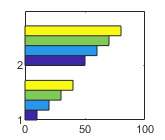`'hist'`

Display the bars in histogram format. Each group is centered at the corresponding `x` value.

Note

A better way to display a horizontal histogram is to call the `histogram` function and specify the `Orientation` name-value pair argument.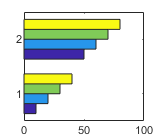Bar color, specified as one of the options in this table.

OptionsDescription
`'blue'` or `'b'`Blue
`'red'` or `'r'`Red
`'green'` or `'g'`Green
`'cyan'` or `'c'`Cyan
`'magenta'` or `'m'`Magenta
`'yellow'` or `'y'`Yellow
`'black'` or `'k'`Black
`'white'` or `'w'`White

Example: `barh([10 20 30],'r')` creates a set of red bars.

Target axes, specified as an `Axes` object. If you do not specify the axes, then the bar graph displays in the current axes.

### Name-Value Arguments

Specify optional comma-separated pairs of `Name,Value` arguments. `Name` is the argument name and `Value` is the corresponding value. `Name` must appear inside quotes. You can specify several name and value pair arguments in any order as `Name1,Value1,...,NameN,ValueN`.

Example: `barh([10 20 30],'EdgeColor','g')` specifies a green outline around the bars.

Note

• The properties listed here are only a subset. For a complete list, see Bar Properties.

• You can set these properties only on bar graphs that use the default `'grouped'` or `'stacked'` style.

Outline color, specified as `'flat'`, an RGB triplet, a hexadecimal color code, a color name, or a short name. If there are 150 bars or fewer, the default value is `[0 0 0]`, which corresponds to black. If there are more than 150 adjacent bars, the default value is `'none'`.

Starting in R2017b, the `'flat'` option uses the `CData` values to color the edges. In previous releases, the `'flat'` option colored the edges using colors from the colormap.

For a custom color, specify an RGB triplet or a hexadecimal color code.

• An RGB triplet is a three-element row vector whose elements specify the intensities of the red, green, and blue components of the color. The intensities must be in the range `[0,1]`; for example, ```[0.4 0.6 0.7]```.

• A hexadecimal color code is a character vector or a string scalar that starts with a hash symbol (`#`) followed by three or six hexadecimal digits, which can range from `0` to `F`. The values are not case sensitive. Thus, the color codes `'#FF8800'`, `'#ff8800'`, `'#F80'`, and `'#f80'` are equivalent.

Alternatively, you can specify some common colors by name. This table lists the named color options, the equivalent RGB triplets, and hexadecimal color codes.

Color NameShort NameRGB TripletHexadecimal Color CodeAppearance
`'red'``'r'``[1 0 0]``'#FF0000'``'green'``'g'``[0 1 0]``'#00FF00'``'blue'``'b'``[0 0 1]``'#0000FF'``'cyan'` `'c'``[0 1 1]``'#00FFFF'``'magenta'``'m'``[1 0 1]``'#FF00FF'``'yellow'``'y'``[1 1 0]``'#FFFF00'``'black'``'k'``[0 0 0]``'#000000'``'white'``'w'``[1 1 1]``'#FFFFFF'``'none'`Not applicableNot applicableNot applicableNo color

Here are the RGB triplets and hexadecimal color codes for the default colors MATLAB® uses in many types of plots.

`[0 0.4470 0.7410]``'#0072BD'``[0.8500 0.3250 0.0980]``'#D95319'``[0.9290 0.6940 0.1250]``'#EDB120'``[0.4940 0.1840 0.5560]``'#7E2F8E'``[0.4660 0.6740 0.1880]``'#77AC30'``[0.3010 0.7450 0.9330]``'#4DBEEE'``[0.6350 0.0780 0.1840]``'#A2142F'`Example: `b = bar(1:10,'EdgeColor','red')`

Example: `b.EdgeColor = [0 0.5 0.5];`

Example: `b.EdgeColor = 'flat';`

Example: `b.EdgeColor = '#D2F9A7';`

Fill color, specified as `'flat'`, an RGB triplet, a hexadecimal color code, a color name, or a short name. The `'flat'` option uses the `CData` property value of the `Bar` object to color the faces.

For a custom color, specify an RGB triplet or a hexadecimal color code.

• An RGB triplet is a three-element row vector whose elements specify the intensities of the red, green, and blue components of the color. The intensities must be in the range `[0,1]`; for example, ```[0.4 0.6 0.7]```.

• A hexadecimal color code is a character vector or a string scalar that starts with a hash symbol (`#`) followed by three or six hexadecimal digits, which can range from `0` to `F`. The values are not case sensitive. Thus, the color codes `'#FF8800'`, `'#ff8800'`, `'#F80'`, and `'#f80'` are equivalent.

Alternatively, you can specify some common colors by name. This table lists the named color options, the equivalent RGB triplets, and hexadecimal color codes.

Color NameShort NameRGB TripletHexadecimal Color CodeAppearance
`'red'``'r'``[1 0 0]``'#FF0000'``'green'``'g'``[0 1 0]``'#00FF00'``'blue'``'b'``[0 0 1]``'#0000FF'``'cyan'` `'c'``[0 1 1]``'#00FFFF'``'magenta'``'m'``[1 0 1]``'#FF00FF'``'yellow'``'y'``[1 1 0]``'#FFFF00'``'black'``'k'``[0 0 0]``'#000000'``'white'``'w'``[1 1 1]``'#FFFFFF'``'none'`Not applicableNot applicableNot applicableNo color

Here are the RGB triplets and hexadecimal color codes for the default colors MATLAB uses in many types of plots.

`[0 0.4470 0.7410]``'#0072BD'``[0.8500 0.3250 0.0980]``'#D95319'``[0.9290 0.6940 0.1250]``'#EDB120'``[0.4940 0.1840 0.5560]``'#7E2F8E'``[0.4660 0.6740 0.1880]``'#77AC30'``[0.3010 0.7450 0.9330]``'#4DBEEE'``[0.6350 0.0780 0.1840]``'#A2142F'`Starting in R2017b, the default value is an RGB triplet from the `ColorOrder` property of the axes. In previous releases, the default value was `'flat'` and the colors were based on the colormap.

Example: `b = bar(1:10,'FaceColor','red')`

Example: `b.FaceColor = [0 0.5 0.5];`

Example: `b.FaceColor = 'flat';`

Example: `b.FaceColor = '#D2F9A7';`

Width of bar outlines, specified as a positive value in point units. One point equals 1/72 inch.

Example: `1.5`

Data Types: `single` | `double` | `int8` | `int16` | `int32` | `int64` | `uint8` | `uint16` | `uint32` | `uint64`

Baseline value, specified as a numeric scalar value.

The baseline value that you specify applies to either the x-axis or the y-axis depending on the bar chart orientation. If you change the orientation of the bar chart from vertical to horizontal, or vice versa, the baseline value might change. Set the `BaseValue` property after setting the `Horizontal` property.

## Output Arguments

collapse all

`Bar` objects. Use the elements in `b` to access and modify properties of a specific `Bar` object after it has been created. The number of `Bar` objects depends on the size of `y`. If `y` is a vector, then `b` is one `Bar` object. If `y` is a matrix, then `b` is a vector containing a `Bar` object for each series in `y`.

collapse all

### Series of Bars

A series consists of the bars at all locations in `X` for a particular set of data. By default, each series of bars is indicated by a different color.

### Group of Bars

A group consists of all the bars at a particular location in `X`.

## Extended Capabilities

### External Websites

Introduced before R2006a